Life In 19x19http://lifein19x19.com/ This 'n' thathttp://lifein19x19.com/viewtopic.php?f=12&t=12327 Page 6 of 40

 Author: Bill Spight [ Sat Nov 14, 2015 11:30 am ] Post subject: Re: This 'n' that Sente or gote? Click Here To Show Diagram Code`[go]\$\$ Outer stones alive\$\$ . . . . . . .\$\$ . . X X X . .\$\$ . . X . X . .\$\$ . . O X O . .\$\$ . . O X O . .\$\$ . . O O O . .\$\$ . . . . . . .[/go]`This kind of play has been described in the literature in two ways, as either a 1 point sente or a 2 point gote (by swing values). Let’s analyze it in terms of play in an environment with temperature 1, which fits either case. Click Here To Show Diagram Code`[go]\$\$B Black first\$\$ . . . . . . .\$\$ . . X X X . .\$\$ . . X 1 X . .\$\$ . . O X O . .\$\$ . . O X O . .\$\$ . . O O O . .\$\$ . . . . . . .[/go]`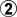elsewhereThe local result is 0, and the net result afteris -1, as advertised.Next let’s look at it as a White sente. Click Here To Show Diagram Code`[go]\$\$W White sente\$\$ . . . . . . .\$\$ . . X X X . .\$\$ . . X 1 X . .\$\$ . . O B O . .\$\$ . . O X O . .\$\$ . . O O O . .\$\$ . . . . . . .[/go]`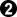takes back at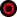The result is -1. Sente gains nothing.Click Here To Show Diagram Code`[go]\$\$W White gote\$\$ . . . . . . .\$\$ . . X X X . .\$\$ . . X 1 X . .\$\$ . . O B O . .\$\$ . . O B O . .\$\$ . . O O O . .\$\$ . . . . . . .[/go]`takes thestones, leaving Click Here To Show Diagram Code`[go]\$\$W White gote, ii\$\$ . . . . . . .\$\$ . . X X X . .\$\$ . . X W X . .\$\$ . . O . O . .\$\$ . . O . O . .\$\$ . . O O O . .\$\$ . . . . . . .[/go]`The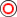stone is en prise. Now we know already that this position is gote with a play gaining 1 point. Let’s continue play at temperature 1. Click Here To Show Diagram Code`[go]\$\$W White gote, iii\$\$ . . . . . . .\$\$ . . X X X . .\$\$ . . X O X . .\$\$ . . O 3 O . .\$\$ . . O C O . .\$\$ . . O O O . .\$\$ . . . . . . .[/go]`,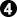elsewhereAfter, unlike with prototypical gote, the play at temperature 1 is not over. So White connects at 3.White has two points for the captured stones plus one point of territory (marked), and Black has two points elsewhere, for a net result of -1.White does not have the privilege of playingwith sente. OTOH, Black may will reply toas it does not lower the local temperature, so it will often be played with sente. Sente or gote?For a long time I did not care.Then for a while I leaned towards sente. But without privilege, Black could often get the reverse sente in normal play. Finally I admitted that it lies on the cusp of sente and gote, and introduced the new classification of ambiguous. Also, I realized that other kinds of plays could be ambiguous, those in which a player had the privilege of playing one option with sente or playing another with gote, leading to the same evaluation. See http://senseis.xmp.net/?AmbiguousPosition on Sensei’s Library.Author: Bill Spight [ Sun Nov 15, 2015 9:26 pm ] Post subject: Re: This 'n' that The reverse Click Here To Show Diagram Code`[go]\$\$ Outer stones alive\$\$ . . . . . . .\$\$ . . X X X . .\$\$ . . X . X . .\$\$ . . O . O . .\$\$ . . O B O . .\$\$ . . O O O . .\$\$ . . . . . . .[/go]`I’m getting a lot of mileage out of these short corridors, aren’t I?I suppose that an inexperienced player might look at this position and just see a dead Black stone. Let’s look more closely. Click Here To Show Diagram Code`[go]\$\$W White first\$\$ . . . . . . .\$\$ . . X X X . .\$\$ . . X . X . .\$\$ . . O 1 O . .\$\$ . . O X O . .\$\$ . . O O O . .\$\$ . . . . . . .[/go]`elsewhereResult: t0 - 2 Click Here To Show Diagram Code`[go]\$\$B Black first\$\$ . . . . . . .\$\$ . . X X X . .\$\$ . . X . X . .\$\$ . . O 1 O . .\$\$ . . O B O . .\$\$ . . O O O . .\$\$ . . . . . . .[/go]`moves to the position in the previous note. We already know that v1 = -1 andt1 = 1.On the assumption thatis gote, we havet0 - 2 = -1 - t0and sot0 = 0.5In that case, t1 > t0, so play continues at temperature 1. Click Here To Show Diagram Code`[go]\$\$B Black first\$\$ . . . . . . .\$\$ . . X X X . .\$\$ . . X 2 X . .\$\$ . . O 1 O . .\$\$ . . O B O . .\$\$ . . O O O . .\$\$ . . . . . . .[/go]`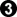takes back at 1,elsewhereThe local score is -1, so we still have t0 = 0.5, and the original count is -1.5. The play by White gains 0.5, and the sequence,-,, also gains 0.5. We consider that sequence as a unit.Experienced players are familiar with sequences of play that end in gote that are played as a unit. However, there is not yet a generally accepted term for those sequences. Combinatorial game theory callsin this position a reversible play, so I propose to call the gote sequence a reverse.Here are a couple of other examples. Click Here To Show Diagram Code`[go]\$\$B Honinbo Shusai Meijin (w) vs. Go Seigen (3 stones)\$\$ ---------------------------------------\$\$ | . . . . . . . 3 1 2 . O O X . . . X . |\$\$ | . X X . . . X X O O O O X X . X X O . |\$\$ | . O X . X X X O O X X . X . . X O O O |\$\$ | . . O X . O O X O , . . X . . X X X O |\$\$ | . . O X . X . X O X X O O X X . . O . |\$\$ | X X X . O . X . O . X O O O O . O . . |\$\$ | X O X . O X X X O O X X O X X O . O . |\$\$ | O O X X X O X O O X X O O X . X O . O |\$\$ | . O X . O O O O . O O X X X X X X O . |\$\$ | . O X , O X O X O , X O O O X X X X O |\$\$ | . . O X O X O X O . . O . O X . . X . |\$\$ | . . O X X X O . . . . . O O O X X . X |\$\$ | . O X X O O O O . . . O X X O X X X . |\$\$ | . . O X X X O . O . . O X O . O O X . |\$\$ | . O O X . X O O X O O X X O . . O X . |\$\$ | . O X X . O X X X , O . X X X X X . . |\$\$ | . . O X . O X . X O . O X O O O X . . |\$\$ | . . O O X O X . X O O . O . . O O X X |\$\$ | . . . O X X . 5 4 6 . . . . . . . O . |\$\$ ---------------------------------------[/go]`Here are the final plays from a three stone game between Shusai and Go Seigen in 1929.-and-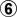are both reverses called hane-and-connect. Each reverse gains 1 point. Click Here To Show Diagram Code`[go]\$\$B Honinbo Retsugen (W) vs. Yasui Chitoku\$\$ ---------------------------------------\$\$ | . . . . . . X O O O . . . . O X . . . |\$\$ | . . . . . . X X O . O . . . O X X . . |\$\$ | . . . X X X . X X O O . . . O X . X . |\$\$ | . X X O X O O X X X O . . O O X X . . |\$\$ | . X O O O . O X . O O . O . O X . . X |\$\$ | X O O X X . O O X O X . O . O X X X O |\$\$ | X . O . . O . . X X O . O O . O O O O |\$\$ | X O O X X X O . X O O O X O O . . X O |\$\$ | . X X X O . O . . O X X X X X X X X O |\$\$ | . . X X O . O X X X O . . X O O O O . |\$\$ | X X O X X O X O . X X X . X X O O . . |\$\$ | X O O O O O X X X . . X X O O X X O . |\$\$ | X X O . O X X O . . O X O X O . . . . |\$\$ | X O O . O O O X X X X O O X . . O O . |\$\$ | O . . O X X X X X O . O O O O O O X O |\$\$ | . . . O X X O O X O . O X X X X O X . |\$\$ | . O . O X O . O X O . X . . . X X . X |\$\$ | . . O . X O O O O O X X X . . . . X . |\$\$ | . 2 1 3 X X O . . O O O X . . . . . . |\$\$ ---------------------------------------[/go]`Here are the final three moves from a castle game between Honinbo Retsugen and Yasui Chitoku in 1802. This is a common reverse to gain 0.5 point. Click Here To Show Diagram Code`[go]\$\$W Honinbo Genjo (W) vs. Yasui Chitoku\$\$ ---------------------------------------\$\$ | . . X O . . . . . . . . . X . . . . . |\$\$ | . X X O . . . . . . . O X . X . . . . |\$\$ | . X O O . O O O . O O O O X X . . . . |\$\$ | . X O , O X X O . , X X X . . , X . . |\$\$ | X X X . X X O X X X . . . X . . . . . |\$\$ | . O X X X O O O X O O . O . . X . . . |\$\$ | . O O O O . . X . X O . O X X O X . . |\$\$ | . . . . . . O X X X O . . O O O X . . |\$\$ | . O O . O . O O . O . . . O O X X . . |\$\$ | . O X O . X O X O , . . O X O , X . . |\$\$ | O O X . O O X X X O . X O X O X . X . |\$\$ | O X X . O X X X X . O O O X X . X O . |\$\$ | X . X O . O X . X O . . X X X X X O . |\$\$ | . X . X O . O X . X O O X O . X O . . |\$\$ | . . . X O O . . X X O X O O 5 X O . . |\$\$ | . . X , O X O . X O X X 4 2 1 X O . . |\$\$ | . . . X O . O . X . X O O 3 X O X O . |\$\$ | . . X X O . . O X X X X O . O O . . . |\$\$ | . . X O O . . . . . . O . . . . . . . |\$\$ ---------------------------------------[/go]`From another Chitoku game in 1802.-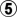is a 5 move reverse that gains 1.5 points.Here is Black's play. Click Here To Show Diagram Code`[go]\$\$B Black's gote\$\$ ---------------------------------------\$\$ | . . X O . . . . . . . . . X . . . . . |\$\$ | . X X O . . . . . . . O X . X . . . . |\$\$ | . X O O . O O O . O O O O X X . . . . |\$\$ | . X O , O X X O . , X X X . . , X . . |\$\$ | X X X . X X O X X X . . . X . . . . . |\$\$ | . O X X X O O O X O O . O . . X . . . |\$\$ | . O O O O . . X . X O . O X X O X . . |\$\$ | . . . . . . O X X X O . . O O O X . . |\$\$ | . O O . O . O O . O . . . O O X X . . |\$\$ | . O X O . X O X O , . . O X O , X . . |\$\$ | O O X . O O X X X O . X O X O X . X . |\$\$ | O X X . O X X X X . O O O X X . X O . |\$\$ | X . X O . O X . X O . . X X X X X O . |\$\$ | . X . X O . O X . X O O X O . X O . . |\$\$ | . . . X O O . . X X O X O O 5 X O . . |\$\$ | . . X , O X O . X O X X 4 3 2 X O . . |\$\$ | . . . X O . O . X . X O O 1 X O X O . |\$\$ | . . X X O . . O X X X X O 6 O O . . . |\$\$ | . . X O O . . . . . . O . . . . . . . |\$\$ ---------------------------------------[/go]`at 2.Note that this is also a reverse.Reverses are fairly common, and recognizable. But sometimes they can fool even an experienced calculator. I have seen it happen. I do not recall ever getting caught, myself, but way back when I paid more attention to the count than the local temperature, and I probably did goof once or twice.Author: Bill Spight [ Wed Nov 18, 2015 4:39 pm ] Post subject: Re: This 'n' that Here is a study based on my research way back when.Black to play. 6.5 komi.Comments?Enjoy!Author: Shaddy [ Wed Nov 18, 2015 5:08 pm ] Post subject: Re: This 'n' that What's the ruleset?

 Author: Bill Spight [ Wed Nov 18, 2015 5:17 pm ] Post subject: Re: This 'n' that Shaddy wrote:What's the ruleset?Territory.Author: Bill Spight [ Fri Nov 20, 2015 10:17 am ] Post subject: Re: This 'n' that OK. Let's calculate the size of plays in the study.Click Here To Show Diagram Code`[go]\$\$B Black first\$\$ ---------------------------\$\$ | . . . . . X C W W 2 1 W C |\$\$ | X X . . . X C W X O X X X |\$\$ | O X X . . X X X X O O X O |\$\$ | O O X , X . X O O O X X . |\$\$ | . O O X . X O . . O O X . |\$\$ | . 2 1 3 X O O . O O X X . |\$\$ | . . O . X O X . O X X . . |\$\$ | . . O X X O O O . 1 O X X |\$\$ | . . O X . X O . X . O O X |\$\$ | . O O O X . X O O O . . O |\$\$ | . O X X X X . X O . O O . |\$\$ | O X . C . . . X X O . . . |\$\$ | . 1 C C . . . C 3 1 2 . . |\$\$ ---------------------------[/go]`The top right is Black's 3 point sente. The threat gains 4 points.In the bottom leftmakes 3 points for Black, and the hane/connect in the bottom right makes 1 point. The other plays make no points. To calculate the gote we need to see the results if White plays first, as well. Click Here To Show Diagram Code`[go]\$\$W White first\$\$ ---------------------------\$\$ | . . . . . X . O O . 1 O . |\$\$ | X X . . . X . O X O X X X |\$\$ | O X X . . X X X X O O X O |\$\$ | O O X , X . X O O O X X . |\$\$ | . O O X . X O . . O O X . |\$\$ | . C 1 . X O O . O O X X . |\$\$ | . . O . X O X . O X X . . |\$\$ | . . O X X O O O C 1 O X X |\$\$ | . . O X . X O C B C O O X |\$\$ | . O O O X . X O O O . . O |\$\$ | C O X X X X . X O . O O . |\$\$ | O B 1 2 . . . X X O . . . |\$\$ | C 3 7 6 . . . 2 1 3 C . . |\$\$ ---------------------------[/go]`saves the stone in the top right corner.-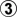captures thestone in the bottom left corner. Later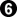is ambiguous, but we count it like a sente. With the captured stone White makes 4 points locally. The swing is 7 points. Each play gains 3.5 points.in the center right makes 5 points. Each play gains 2.5 points.The hane/connect in the bottom right makes 1 point. Each play gains 1 point.The connection on the left side makes 1 point. Each play gains 0.5 point.These calculations give us a possible order of play, from the largest play to the smallest: Bottom left, top right, center right, bottom right, left side. That is the main line in the SGF file below. Black wins by 0.5.But wait! The top right is not only a local sente, it is global sente, since its threat is greater than the bottom left gote. Shouldn't Black play it first? Isn't it the right play, even if it does not change the score?When I was doing this research, I remembered how, when I first started playing over pro games, as the game wound down, they would sometimes make haste to take their sente plays, regardless of the size of the sente. I figured it was because they knew they would not need them for ko threats, and did not want them to get away. And indeed, you can show with CGT (combinatorial game theory) that when there are no kos about, taking your global sente loses nothing to par. (I didn't know CGT at the time, but that was still obvious.)Variation 1 shows the result after Black plays sente in the top right and White answers. (There is a side variation when White mistakenly does not answer the sente. I included it because I think that the later play is of some interest.) Black then takes the gote in the bottom left. Curiously, White wins by 0.5.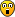Well, the sente may lose nothing to par, but, as the great bridge writer Victor Mollo said, par is a poor target.On the theory that it is easy to compare simple gote, this is the rule that I came up with to decide whether to play global sente or not, in an environment of simple gote.Let r be the value of the reverse sente, and t0 ≥ t1 ≥ t2 ≥ . . . be how much the gote gain. t0 is the temperature of the environment.If r < 2t1 - 2t2 + 2t3 - . . .then do not take the sente, but take the largest gote. Note that t0 does not appear in this comparison, because the sente player gets it in either case. Since it is not part of the comparison, it is best not to consider it part of the environment, either, and consider t1 to be the ambient temperature.In this case, 3 < 5 - 2 + 1 = 4, so my rule says to play in the bottom left. And indeed, if Black plays the sente in the top right, a loss by 0.5 is fairly predictable.So is a 0.5 point loss the par result? Unfortunately, yes. In the main line White erred by playing the reverse sente. See the variation at move 2 for the correct play. White takes the theoretically smaller gote and wins by 0.5. The reason that the theoretically smaller play is correct has to do with the drop in the ambient temperature after it is played. If White takes the reverse sente he gains 3 points but then loses 2 points for a net gain of 1 point, while if he takes the gote he gains 2 points, which is 1 point better. We could say that by taking the gote White gets the last play at temperature 2.5. The whole point of getting the "last play" has to do with the subsequent drop in temperature. There is nothing magical about the last play itself, or the last play of the opening, or the last play of the large endgame. It is the temperature drop that is important.Now, I was already aware the the best move is not always the theoretically largest play. In fact, in my research I was trying to find simple rules that would tell me when not to play the theoretically largest play. Although this example is somewhat interesting and instructive, my rule does not quite fit the bill. Why not?Because it is tantamount to reading the whole board out. To be practical, this kind of rule needs to be approximate, so that you don't have to read the whole board out. That is why the environment consists of simple gote. Few real environments consist of simple gote, but they are approximately like that. That is why the largest play is very likely to be the best play. With approximations, the rule is really a set of possible rules:r < t1r < 2t1 - t2r < 2t1 - 2t2 + t3* * *But with approximations come possible errors. In this case we know that playing the global sente is never technically incorrect. It is just that playing the largest gote might induce an error by our opponent. But if the rule is approximate, playing the largest gote might be an error itself.

 Author: Schachus [ Fri Nov 20, 2015 10:23 am ] Post subject: Re: This 'n' that I was just about to post this:Not, sure whether this is sensible, so probably no point in hiding... but anyway: for me, the main question seems to be whether 1-3 in the upper right is sente for b. It seems to be a 4 point gote with an 8 pnot gote followup, so that the miai value(gain) of b's move is 4 both when treated as sente or gote. But I'm not clever enough to find the temerature of the remaining board(I guess it's 4 because you likely constructed it that way. The other big spot seems to be 2-1 in the lower left(respecively cut from w's perspective), but I didnt count how big that is(edit: it seems to be a little more than 7 points gote(that is 7 if black later gets the 1-4 in sente, which seems likely to me), so a little, but not much less than 4 points. Still that doesnt really explain why white should tenuki the 8point gote in top right, to take lower left...). My guess is that b should try to get upper right in sente and then lower left, which is probably why white needs to consider to treat upper right as gote and take lower left(edit: the count of lower left doesnt really back that up...), if that is too good for w , that would mean b has to start here. Maybe I'll try to play this to the end and count it later.)would already be happy if this is even the right directionEdit after I read some of your post: the largerst error seems to have been that I thought top right was 4 points gote with 8 points followup, while it really is is only 3 points, and thus the followup is big enough to make it sente.

 Author: ez4u [ Sat Nov 21, 2015 5:45 pm ] Post subject: Re: This 'n' that It seems like Black has gotten overconfident in the last line. White ignores 11 to take the last point and will be able to win the ko handily with threats at a, b(2), and c. Black has only the threats at d and e. Instead of 7, Black should play the hane in the lower right at 10, gaining ko threats. When White plays the hane at 7, Black can now win the ko so it will be gote and Black gets the last point. Am I missing something?Click Here To Show Diagram Code`[go]\$\$Bcm7\$\$ ---------------------------\$\$ | . . . . . X . O O X X O . |\$\$ | X X . . . X . O X O X X X |\$\$ | O X X . . X X X X O O X O |\$\$ | O O X c X . X O O O X X . |\$\$ | . O O X . X O . . O O X . |\$\$ | . . . 6 X O O . O O X X . |\$\$ | . . O e X O X . O X X b . |\$\$ | . . O X X O O O . O O X X |\$\$ | . . O X . X O . X . O O X |\$\$ | . O O O X . X O O O . . O |\$\$ | . O X X X X a X O . O O d |\$\$ | O . O X . . . X X O . . . |\$\$ | . O 5 1 . . . 3 2 4 . . . |\$\$ ---------------------------[/go]`

 Author: Bill Spight [ Sun Nov 22, 2015 8:18 am ] Post subject: Re: This 'n' that ez4u wrote:It seems like Black has gotten overconfident in the last line. White ignores 11 to take the last point and will be able to win the ko handily with threats at a, b(2), and c. Black has only the threats at d and e. Instead of 7, Black should play the hane in the lower right at 10, gaining ko threats. When White plays the hane at 7, Black can now win the ko so it will be gote and Black gets the last point. Am I missing something?Click Here To Show Diagram Code`[go]\$\$Bcm7\$\$ ---------------------------\$\$ | . . . . . X . O O X X O . |\$\$ | X X . . . X . O X O X X X |\$\$ | O X X . . X X X X O O X O |\$\$ | O O X c X . X O O O X X . |\$\$ | . O O X . X O . . O O X . |\$\$ | . . . 6 X O O . O O X X . |\$\$ | . . O e X O X . O X X b . |\$\$ | . . O X X O O O . O O X X |\$\$ | . . O X . X O . X . O O X |\$\$ | . O O O X . X O O O . . O |\$\$ | . O X X X X a X O . O O d |\$\$ | O . O X . . . X X O . . . |\$\$ | . O 5 1 . . . 3 2 4 . . . |\$\$ ---------------------------[/go]`Thanks, Dave!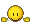I got careless, didn't I?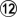is a slight inaccuracy. The solid connection is technically better. White still wins, though.Click Here To Show Diagram Code`[go]\$\$Bcm13 Ko fight\$\$ ---------------------------\$\$ | . . . . . X . O O X X O . |\$\$ | X X . . . X . O X O X X X |\$\$ | O X X 3 . X X X X O O X O |\$\$ | O O X 2 X . X O O O X X . |\$\$ | . O O X . X O . . O O X . |\$\$ | . . . O X O O . O O X X . |\$\$ | . . O . X O X . O X X . . |\$\$ | . . O X X O O O . O O X X |\$\$ | . . O X . X O . X . O O X |\$\$ | . O O O X . X O O O . 6 O |\$\$ | . O X X X X 8 X O . O O 5 |\$\$ | O 1 O X . . 9 X X O . . . |\$\$ | . O X X . . . X O O . . . |\$\$ ---------------------------[/go]`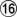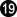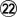take ko. Click Here To Show Diagram Code`[go]\$\$Bcm23 Ko fight continued\$\$ ---------------------------\$\$ | . . . . . X . O O X X O . |\$\$ | X X . . . X . O X O X X X |\$\$ | O X X X . X X X X O O X O |\$\$ | O O X . X . X O O O X X . |\$\$ | . O O X . X O . . O O X . |\$\$ | . 2 1 O X O O . O O X X 0 |\$\$ | . . O 7 X O X . O X X 4 5 |\$\$ | . . O X X O O O . O O X X |\$\$ | . . O X . X O . X . O O X |\$\$ | . O O O X . X O O O . O O |\$\$ | . O X X X X . X O . O O X |\$\$ | O 3 W X . . X X X O . . . |\$\$ | . O X X . . . X O O . . . |\$\$ ---------------------------[/go]`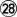takes ko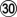fills ko at 25.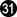takes ko at 23.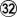is the winning ko threat.Thanks again, Dave. A great example of komonster.Author: Krama [ Sun Nov 22, 2015 3:03 pm ] Post subject: Re: This 'n' that What the hell did I just read? (last few posts)This is more math than go.What are these calculations?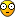??

 Author: ez4u [ Sun Nov 22, 2015 4:23 pm ] Post subject: Re: This 'n' that Bill Spight wrote:ez4u wrote:... Am I missing something?Click Here To Show Diagram Code`[go]\$\$Bcm7\$\$ ---------------------------\$\$ | . . . . . X . O O X X O . |\$\$ | X X . . . X . O X O X X X |\$\$ | O X X . . X X X X O O X O |\$\$ | O O X c X . X O O O X X . |\$\$ | . O O X . X O . . O O X . |\$\$ | . . . 6 X O O . O O X X . |\$\$ | . . O e X O X . O X X b . |\$\$ | . . O X X O O O . O O X X |\$\$ | . . O X . X O . X . O O X |\$\$ | . O O O X . X O O O . . O |\$\$ | . O X X X X a X O . O O d |\$\$ | O . O X . . . X X O . . . |\$\$ | . O 5 1 . . . 3 2 4 . . . |\$\$ ---------------------------[/go]`Thanks, Dave!I got careless, didn't I?is a slight inaccuracy. The solid connection is technically better. White still wins, though.... A great example of komonster.I think 12 is more than a slight inaccuracy, and very interesting. The 12 in my diagram looks so natural that I gave it no thought even though I was diagramming a ko fight!It looks like it threatens to make another point by a White play at e. However that is never going to happen because e is a ko threat for Black. So all it does is give White one more ko threat at c. But it gives Black two additional ko threats (C8 before e)! White squeaks through here. But if White had only two ko threats to begin with, 12 would give the win back to Black. White 12 at C8 would win here with three ko threats to one and would even win if White had only two ko threats.

 Author: Bill Spight [ Sun Nov 22, 2015 10:57 pm ] Post subject: Re: This 'n' that Krama wrote:What the hell did I just read? (last few posts)This is more math than go.What are these calculations???Thank you for the feedback. I do not mean to aim my remarks at mathematicians rather than go players. OTOH, my own research falls into the category of mathematical go, so please bear with me.The calculations for each play are standard. I use algebra to calculate the size of plays by means of equations involving plays of unknown size in the environment. One reason is that I will make use of the algebra for other purposes later on. If you are used to other ways to calculate the size of plays, you can ignore the algebraic calculations.I use algebraic notation for the comparison of sequences and for the rules I derived way back when. Without the algebra they would not be so easy to describe.Author: Bill Spight [ Sun Nov 22, 2015 11:12 pm ] Post subject: Re: This 'n' that Schachus makes an important observation that I will get back to soon. But for now, something different.Korschelt noted that incomplete game records were often published, and said that good players could complete the games correctly on their own. That may not be so for every incomplete game record that we have, but here is a game record that I have backed up to a point where it is not too hard to finish the game correctly. Several sequences lead to the actual, correct score. All roads may not lead to Rome, but many of them do.Click Here To Show Diagram Code`[go]\$\$Bc Black has just played. White to play.\$\$ ---------------------------------------\$\$ | . . O X X . . . . . . . . . . . . . . |\$\$ | . . O O X . . . . X X X X . . . . . . |\$\$ | . . . O . X . X X O X O O X O . . X X |\$\$ | . . O O . . . X O , O . O X . , X X O |\$\$ | . . X O X . . X O O O O O X . X O O O |\$\$ | . . O X X . 1 O . . . X X X X X O . . |\$\$ | . . O O . . X O . O . X O O X O O . . |\$\$ | . O . O X X O . . . O X X O O . . . . |\$\$ | . O O X . X O . O O . O X . O . . . . |\$\$ | . X X , O X X O X , O O X X X O O . . |\$\$ | . . X X O . . O . O X X O . X X X O . |\$\$ | . X . . X X X . O . . X O . . . X O . |\$\$ | . . X X X O O O . O . X O . X X O . . |\$\$ | . O . O . O X X . X . X O X X O O . . |\$\$ | O O . O . O . . . X X O . O O . . . . |\$\$ | O X X X X O X X O , . O . . O , O . . |\$\$ | X X . O X X O . X X . O . . . . . . . |\$\$ | . . . . . . . . . . X X O O O O . . . |\$\$ | . . . . . . . . . . X X X X X O . . . |\$\$ ---------------------------------------[/go]`White to play. Each side has captured three stones.Enjoy!Author: mitsun [ Mon Nov 23, 2015 11:54 am ] Post subject: Re: This 'n' that Oops, I wrote this up a few days ago, in response to the previous problem, but forgot to post it. Covering old ground now, but with a slightly different counting method, and perhaps a bit less math. (I also neglected the possible ko in the lower left.)The lower left has a gote play worth 7 points (difference counting). The center has a gote play worth 5 points. The lower right has a gote play worth 2 points. The upper left has a gote play worth 1 point.The upper right is a bit more complicated. There is a play for B that gains 3 points immediately and creates an 8 point follow-up. In games like this where the follow-up is large compared to other moves on the board, this play can be evaluated as 3 point sente. In other situations, it might be evaluated as gote 3+(1/2)*8, where the (1/2) is some sort of probability or statistical average over board positions. W might evaluate this as (2)*3 point reverse sente, but again the (2) is only a general estimate. If B starts with the UR play, perhaps realizing that it is sente, then W responds as expected. The remaining gote plays are then taken in order of size. If I counted correctly, B is ahead by only 6 points on the board. This agrees with the count {+3,+7,-5,+2,-1} = +6. If B starts with the LL gote, W should take the large center gote. B can again take the UR play in sente. The remaining gote plays are then taken in order of size. B is again ahead by only 6 points on the board. This agrees with the count {+7,-5,+3,+2,-1} = +6. This is the same as the previous play, just changing the sequence a little.If B starts with the LL gote, it is a mistake for W to take the UR play (perhaps reasoning that it is reverse sente). The remaining gote plays are then taken in order of size. This time B is ahead by 7 points on the board. In some rulesets, I guess W has to pass at the end, giving B one more point. This agrees with the count {+7,-3,+5,-2,+1} = +8.The point I suppose is that it can be incorrect to take a reverse sente play? Otherwise play seems to be simply by order of size. B is free to take the sente immediately or defer it.

 Author: Bill Spight [ Tue Nov 24, 2015 10:01 am ] Post subject: Re: This 'n' that mitsun wrote:Oops, I wrote this up a few days ago, in response to the previous problem, but forgot to post it. Covering old ground now, but with a slightly different counting method, and perhaps a bit less math. (I also neglected the possible ko in the lower left.)The lower left has a gote play worth 7 points (difference counting). The center has a gote play worth 5 points. The lower right has a gote play worth 2 points. The upper left has a gote play worth 1 point.Quite so.Quote:The upper right is a bit more complicated. There is a play for B that gains 3 points immediately and creates an 8 point follow-up. In games like this where the follow-up is large compared to other moves on the board, this play can be evaluated as 3 point sente. In other situations, it might be evaluated as gote 3+(1/2)*8, where the (1/2) is some sort of probability or statistical average over board positions. W might evaluate this as (2)*3 point reverse sente, but again the (2) is only a general estimate. It is true that on some boards by correct play this corner will be played as gote by Black. However, it is classified as a sente, and is counted as three points of territory for Black. The reverse sente gains three points.Quote:If B starts with the UR play, perhaps realizing that it is sente, then W responds as expected. The remaining gote plays are then taken in order of size. If I counted correctly, B is ahead by only 6 points on the board. This agrees with the count {+3,+7,-5,+2,-1} = +6. If B starts with the LL gote, W should take the large center gote. B can again take the UR play in sente. The remaining gote plays are then taken in order of size. B is again ahead by only 6 points on the board. This agrees with the count {+7,-5,+3,+2,-1} = +6. This is the same as the previous play, just changing the sequence a little.If B starts with the LL gote, it is a mistake for W to take the UR play (perhaps reasoning that it is reverse sente). The remaining gote plays are then taken in order of size. This time B is ahead by 7 points on the board. In some rulesets, I guess W has to pass at the end, giving B one more point. This agrees with the count {+7,-3,+5,-2,+1} = +8.Difference counting (what I am calling swing counting) may be useful for comparisons, but it does not tell us what each play gains. Somehow this is seldom taught, leading to much confusion.In the original position Black is ahead by 4.5 points. Then with correct play Black gains 1.5 points for a total of 6 points. With komi White wins by 0.5.Variation 1: Black starts with the sente in the top right. Sente gains nothing, so the board count remains 4.5. Then Black gains 3.5 in the bottom left, for a count of 8. Then White gains 2.5 in the center right, for a count of 5.5. Then Black gains 1 point on the bottom side, for a count of 6.5. Then White gains 0.5 point on the left side, for a board score of 6. As advertised. Variation 2: Black starts with the gote in the bottom left, gaining 3.5 points for a count of 8. then White takes the reverse sente in the top right, gaining 3 points for a count of 5. Then Black gains 2.5 in the center right, for a count of 7.5. Then White gains 1 point on the bottom side, for a count of 6.5. The Black gains 0.5 point on the left side, for a board score of 7. As advertised. To show that the original board count is 4.5, the board score should be 3 if we let White play first, taking the gote while avoiding the ko, and letting Black take the sente in the top right. Click Here To Show Diagram Code`[go]\$\$Wc White first\$\$ ---------------------------\$\$ | . . . . . X . O O 5 4 O . |\$\$ | X X . . . X . O X O X X X |\$\$ | O X X . . X X X X O O X O |\$\$ | O O X , X . X O O O X X . |\$\$ | . O O X . X O . . O O X . |\$\$ | . . . . X O O . O O X X . |\$\$ | . . O . X O X . O X X . . |\$\$ | . . O X X O O O . 6 O X X |\$\$ | . . O X . X O . X . O O X |\$\$ | . O O O X . X O O O . . O |\$\$ | . O X X X X . X O . O O . |\$\$ | O X 1 2 . . . X X O . . . |\$\$ | . 3 . . . . . . . . . . . |\$\$ ---------------------------[/go]` Click Here To Show Diagram Code`[go]\$\$Wcm7 White first\$\$ ---------------------------\$\$ | . . . . . X . O O O X O . |\$\$ | X X . . . X . O X O X X X |\$\$ | O X X . . X X X X O O X O |\$\$ | O O X , X . X O O O X X . |\$\$ | . O O X . X O . . O O X . |\$\$ | . 7 6 8 X O O . O O X X . |\$\$ | . . O . X O X . O X X . . |\$\$ | . . O X X O O O . X O X X |\$\$ | . . O X . X O . X . O O X |\$\$ | . O O O X . X O O O . . O |\$\$ | . O X X X X . X O . O O . |\$\$ | O . O X . . . X X O . . . |\$\$ | . O 5 4 . . . 2 1 3 . . . |\$\$ ---------------------------[/go]`Board score: Black has 22 points in the group spanning the board from top to bottom, plus 10 points on the right, for a total of 32.White has 13 points on the left side plus 6 points in the center and 10 points in the bottom right corner, for a total of 29.Net score: +3. As advertised.Edit: If White is still komonster, which I believe is the case, White can make the ko on the bottom side by playing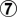at 10, and later forcing Black to take the half point on the left side before gaining one point by filling the ko. That way White gets 2 points on the bottom side, for a net score of +2. The gains and losses still add up:4.5 - 3.5 + 2.5 - 1 + 0.5 - 1 = 2Edit: Actually, to be accurate, it costs White 2/3 point (0.67) to set up the ko. Sente may gain nothing, but it can lose something. Later filling the ko gains 1.67. My mistake above. Here is the corrected accounting:4.5 - 3.5 + 2.5 - 1 + 0.67 + 0.5 - 1.67 = 2

 Author: Bill Spight [ Tue Nov 24, 2015 1:24 pm ] Post subject: Re: This 'n' that Oh, just a note about the gain and loss calculations I'm using. They are traditional. They are not traditional in the sense of going back to 1800, but they were by the time I learned go. In Japanese they are called miai values. O Meien has come up with a new term to refer to them, absolute counting. I just call them gains and losses.Author: Shaddy [ Tue Nov 24, 2015 1:47 pm ] Post subject: Re: This 'n' that Stupid question here - where do 1/3-points come from?

 Author: mitsun [ Tue Nov 24, 2015 4:22 pm ] Post subject: Re: This 'n' that Bill's preferred miai counting calulates points gained per move. The simplest example is the last one-point ko at the end of the game. Suppose B can fill it to end the game, while W has to take it and then fill it to end the game. The total move difference is three moves, for a total point gain of one point, hence each move is worth 1/3 point by miai counting.(Way back when I was learning go, this was generally called a half-point ko. The reasoning was that it was worth one point, and it was a toss-up who would get it at the end of the game. But with a little reflection, you can see that the miai value is more correct mathematically.)(These values are all somewhat statistical in nature. Think of them as the average value or expectation value over many similar positions, across a variety of games with different remaining endgame moves. Of course in any particular game, with a particular best sequence of moves, the actual value will turn out to be 1 point for someone. But if the position is complicated enough that we cannot accurately read who will get that point, then evaluating the local position according to the statistical average can be useful.)

 Author: Bill Spight [ Tue Nov 24, 2015 9:17 pm ] Post subject: Re: This 'n' that Shaddy wrote:Stupid question here - where do 1/3-points come from?Nearly every introductory endgame book has the following diagram. Click Here To Show Diagram Code`[go]\$\$B One point for Black \$\$ . . . . . . . . . . .\$\$ . . X X X X X X X . .\$\$ . . X W X O X O X . . \$\$ . . O . O . O . O . . \$\$ . . O O O O O O O . .\$\$ . . . . . . . . . . .[/go]`The three White ko stones add up to one point for Black, regardless of who plays first. Click Here To Show Diagram Code`[go]\$\$B Black first\$\$ . . . . . . . . . . .\$\$ . . X X X X X X X . .\$\$ . . X W X O X O X . . \$\$ . . O 1 O 2 O 4 O . . \$\$ . . O O O O O O O . .\$\$ . . . . . . . . . . .[/go]`atResult: B +1 Click Here To Show Diagram Code`[go]\$\$W White first\$\$ . . . . . . . . . . .\$\$ . . X X X X X X X . .\$\$ . . X W X O X O X . . \$\$ . . O 2 O 3 O 1 O . . \$\$ . . O O O O O O O . .\$\$ . . . . . . . . . . .[/go]`atResult: B +1In both variations each player made the same number of moves, maintaining the score. The value of each ko is 1/3 point for Black. Filling or taking the ko gains 1/3 point.

 Author: Bill Spight [ Tue Nov 24, 2015 10:27 pm ] Post subject: Re: This 'n' that No bites yet on finishing the endgame. Here are the next 10 moves. Click Here To Show Diagram Code`[go]\$\$Wc White takes his sente\$\$ ---------------------------------------\$\$ | . . O X X . . . . . . . . . . . . . . |\$\$ | . . O O X 2 . . . X X X X . . . . . . |\$\$ | . . . O 1 X . X X O X O O X O . . X X |\$\$ | . . O O 3 4 . X O , O . O X . , X X O |\$\$ | . . X O X . . X O O O O O X . X O O O |\$\$ | . . O X X . X O . . . X X X X X O . . |\$\$ | . . O O 5 6 X O . O . X O O X O O . . |\$\$ | . O . O X X O . . . O X X O O . . . . |\$\$ | . O O X . X O . O O . O X . O . . . . |\$\$ | . X X , O X X O X , O O X X X O O . . |\$\$ | . . X X O . . O . O X X O . X X X O . |\$\$ | . X . . X X X . O . . X O . . . X O . |\$\$ | . . X X X O O O . O . X O . X X O . . |\$\$ | . O . O . O X X . X . X O X X O O . . |\$\$ | O O . O . O . . . X X O . O O . . . . |\$\$ | O X X X X O X X O 0 9 O . . O , O . . |\$\$ | X X . O X X O . X X 7 O . . . . . . . |\$\$ | . . . . . . . . . 8 X X O O O O . . . |\$\$ | . . . . . . . . . . X X X X X O . . . |\$\$ ---------------------------------------[/go]`Plus four more. Click Here To Show Diagram Code`[go]\$\$Wcm11 More White sente\$\$ ---------------------------------------\$\$ | . . O X X . . . . . . . . . . . . . . |\$\$ | . . O O X X . . . X X X X . . . . . . |\$\$ | . . . O O X . X X O X O O X O . . X X |\$\$ | . . O O O X . X O , O . O X . , X X O |\$\$ | . . X O X . . X O O O O O X . X O O O |\$\$ | . . O X X . X O . . . X X X X X O . . |\$\$ | . . O O O X X O . O . X O O X O O . . |\$\$ | . O . O X X O . . . O X X O O . . . . |\$\$ | . O O X . X O . O O . O X . O . . . . |\$\$ | . X X , O X X O X , O O X X X O O . . |\$\$ | . . X X O 4 3 O . O X X O . X X X O . |\$\$ | . X . . X X X . O . . X O . . . X O . |\$\$ | . . X X X O O O . O . X O . X X O . . |\$\$ | . O . O . O X X . X . X O X X O O . . |\$\$ | O O . O . O 1 2 . X X O . O O . . . . |\$\$ | O X X X X O X X O X O O . . O , O . . |\$\$ | X X . O X X O . X X O O . . . . . . . |\$\$ | . . . . . . . . . X X X O O O O . . . |\$\$ | . . . . . . . . . . X X X X X O . . . |\$\$ ---------------------------------------[/go]`What next?

 Page 6 of 40 All times are UTC - 8 hours [ DST ] Powered by phpBB © 2000, 2002, 2005, 2007 phpBB Grouphttp://www.phpbb.com/